# Probability + percentages - math problems

Probability is the measure of the likeliness that an event will occur. The probability (chance) is a value from the interval <0;1> or in percentage (0% to 100%) expressing the occurrence of some event. 0 is impossible event and 1 (100%) means the certainty event.

#### Number of problems found: 50

• Event probability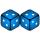The probability of event P in 8 independent experiments is 0.33. What is the probability that the event P occurs in one experiment (chance is the same)?
• Events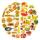Event P has probability of 0.84. What is the probability that the event P occurs in 3, 5, 7 try.
• Shooter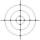The probability that a good shooter hits the center of the target circle I is 0.1. The probability that the target hit intercircle is II 0.58. What is the probability that it hits the target circle I or II?
• Two doctorsDoctor A will determine the correct diagnosis with a probability of 93% and doctor B with a probability of 79%. Calculate the probability of proper diagnosis if both doctors diagnose the patient.
• Bulbs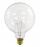The probability that the bulb can operate 4000 hours is 0.3. What is the probability that exactly one of eight bulbs can operate 4000 hours?
• FamilyWhat is the probability that a family with 7 childrens have: exactly 5 girls? 7 girls and 0 boys? Consider the birth probability of a girl is 48.69% and boy 51.31%.
• Probability - tickets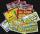What is the probability when you have 25 tickets in 5000 that you not wins the first (one) prize?
• ProbabilityHow probable is a randomly selected three-digit number divisible by five or seven?
• Utopia IslandA probability of disease A on the island of Utopia is 40%. A probability of occurrence among the men of this island, which make up 60% of all the population (the rest are women), is 50%. What is the probability of occurrence of A disease among women on Ut
• Three dice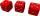What is the probability that when we roll three dice, we roll the numbers 1,2,3?
• Ace or king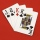What is the probability that we will choose an ace or a king when choosing from a deck of sevens cards?
• Three reds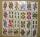What is the probability that when choosing 3 carats from seven carats, all 3 reds will be red?
• Sum 10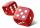What is the probability that two dice throw twice in a row will result the sum of 10?
• DicesWe will throw two dice. What is the probability that the ratio between numbers on first and second dice will be 1:2?
• Win in raffle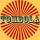The raffle tickets were sold 200, 5 of which were winning. What is the probability that Peter, who bought one ticket will win?
• Three colors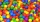Find the probability that 3 balls of the same color will be drawn from fate with 10 white, 10 red, and 10 blue balls.
• Family94 boys are born per 100 girls. Determine the probability that there are two boys in a randomly selected family with three children.
• AceFrom complete sets of playing cards (32 cards), we pulled out one card. What is the probability of pulling the ace?
• Two acesFrom a 32 card box we randomly pick 1 card and then 2 more cards. What is the probability that last two drawn cards are aces?
• ThrowWe throw 2 times with 2 dices. What is the probability that the first roll will fall more than sum of 9 and the second throw have sum 3 or does not have the sum 4?

Do you have an interesting mathematical word problem that you can't solve it? Submit a math problem, and we can try to solve it.

We will send a solution to your e-mail address. Solved examples are also published here. Please enter the e-mail correctly and check whether you don't have a full mailbox.

Please do not submit problems from current active competitions such as Mathematical Olympiad, correspondence seminars etc...